Courses

# Mathematics Notes | EduRev

## Mathematics : Mathematics Notes | EduRev

The document Mathematics Notes | EduRev is a part of the Mathematics Course Algebra for IIT JAM Mathematics.
All you need of Mathematics at this link: Mathematics

Introduction to Relations and Functions
This topic “Relations and Functions” is a foundation or fundamental of algebra in mathematics. Relations and functions are two different words having different meaning mathematically. Many us you might be confused in their difference. We shall study both these concepts in detail here.
Same as the relations which we have in our daily life, a kind of relations also exists in algebra. In daily life, relations are like brother and sister, friends, student and teacher and many more. In mathematics also we see some relations like a line is parallel or perpendicular to another, any one variable is greater or less than the another variable. Any Set A is subset of B, all these are examples of relations.
One thing which we see in common while studying relations, that it required two different objects to link two different objects via relations.

What is the meaning of Relation in math?
Understanding Relations requires basic knowledge of sets. A Set is a collection of well defined objects of particular kind. For Example a set of outcomes of dice, a set of English alphabet.
Relation is always studied between two sets. If we have two non void (or null/empty) sets A and B then the relation R from set A to set B is represented by aRb, where a is the set of elements belonging to set A while b belongs to set B.
Relation from a set A to a set B is the subset of the Cartesian product of A and B i.e. subset of A x B. Relation in other way can also be defined as an collection of ordered pairs (a, b) where a belongs to the elements from set A and b from set B and the relation is from A to B but not vice versa.

For Example
Consider a set A containing elements as {1, 2, 3} and set B contains elements as {2, 4, 6}.
Then the relation between Set A and B from A to B will be set of any combinations from Set A to set B.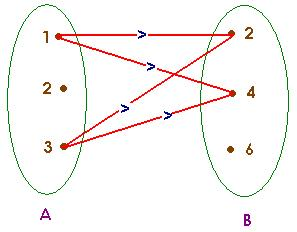From the above diagram, we can see that Relation from A to B i.e. R will be set of {(1,4). (1,2), (3,4), (3,2)}. This relation is a subset of the Cartesian product of two sets A X B.
Let’s take another example where, set A = {1, 2, 3} and set B = {1, 2, 3, 4, 5, 6, 7, 8, 9}.
If the Relation between A and B is as: elements of B is the squares of elements of set A, then the relation is written in the form of sets as:
R  = {(a,b): where b is square of a and a ∈ A & b ∈ B}
Then R = {(1,1), (2,4), (3,9)}
Same as sets, relation may also be represented algebraically either by the Roster method or by the Set-builder method.
Relation can also be defined as a linear operation which establishes relationship between the element’s of two set’s according to some definite rule of relationship.
R : {(a, b) | (a, b) ∈ A x B and a R b}
Eg: 1 A is {2, 3, 5}
B is {1, 4, 9, 25, 30}
If  a R b → b is square of a
Discrete element of relation are {(2, 4), (3, 9). (5, 25)}
Eg: 2  A = {Jaipur, Lucknow, Kanpur, Bhopal}
B = {Rajasthan, Uttar pradesh, Madhya Pradesh}
aRb → a is capital of b,
A X B = {(Jaipur, Rajasthan), (Lucknow, Uttar Pradseh), (Bhopal, Madhya Pradesh}

Total Number of Relation from A to B
Let the number of relations from A to B be x.
Let A contain ‘m’ elements and B contains ‘n’ elements
Number of element’s in A x B → m x n
Number of non void subset’s =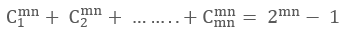Thus, for A = {1, 2, 3)  & B = {x, y, z}
Number of non void subset’s or the number of possible relations = =29 - 1 = 511

What are Functions?
Functions are the special class of relation or we can say that special types of relations are called as Functions. Function is one of the most important concepts in mathematics as every situation in real life are solved and analysed first by writing its mathematical equation or function.
A function is like a machine which gives unique output for each input that is fed into it. But every machine is designed for certain defined inputs for e.g. a washing machine is designed for washing cloths and not the wood. Similarly the functions are defined for certain inputs which are called Functionsas its domain and corresponding outputs are called Range.Let A and B be two sets and let there exist a rule or manner or correspondence ‘f’ wihch associates to each element of A to a unique element in B, then f is called a Function or Mapping from A to B. It is denoted by symbol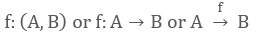Which reads ‘f is a function from A to B' or' f maps A to B.
If an elements a ∈ A is associated with an element b ∈ B then b is called ‘the f image of a’ or ‘image of a under f' or ‘the value of the function f at a’. Also a is called the pre – image of b or argument of b under the function f. we write it as
f:(a, b)  or f:a → b or b = f(a)
A relation f from a set A to a set B is called as the function if it satisfies the below conditions:

• All the elements of A should be mapped with the elements of B. That is, there should not be any element in A which is being unmapped with B.

i.e. ∀a, (a, f(a)) ∈ f, where, a is the elements of set A

• Elements of set A should be uniquely mapped with the elements of set B.

i.e. if (a, b) ∈ f & (a, c) ∈f, ⇒ b = c
Thus the ordered pairs of f must satisfy the property that each element of A appears in some ordered pair and no two ordered pairs have same first elements.
See the below figures to understand the above points.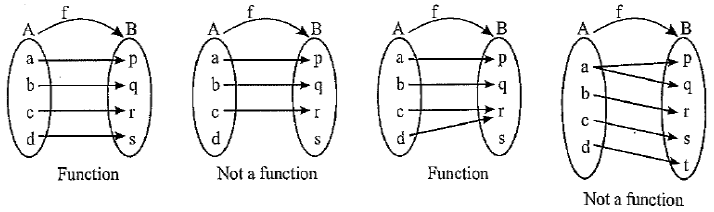Note: Every function is a relation but every relation is not necessarily a function.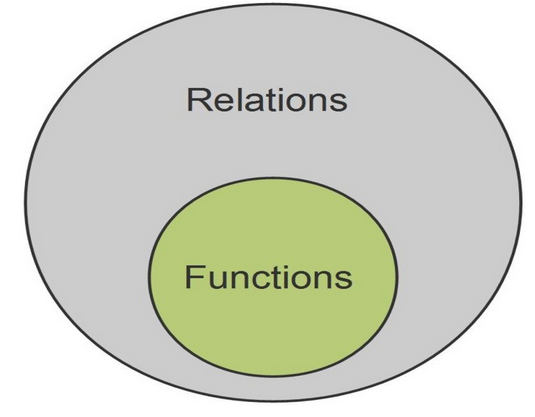What is the domain of a function? Also explain its co-domain and Range
For a relation from set A to set B i.e. aRb, all the elements of set A are called as the domain of the relation R while all the elements of set B are called as the co-domain of the relation R.
Range is the set of all second elements from the ordered pairs (a, b) in the relation aRb.
Domain of f = {a | a ∈ A, (a, f(a)) ∈ f }
Range of f = {f (a) |a ∈ A, f(a) ∈ B, (a, f (a)) ∈ f}
For the relation aRb, domain is considered as the input to relation R while the co-domain is the possible outputs and range is the actual output.
Similarly for a function, f: A → B,  elements of set A are the inputs and B is the set of possible outputs. But the second elements of all ordered pair of f(A, B) will be the actual outputs.
It should be noted that range is a subset of co-domain. If only the rule of function is given then the domain of the function is the set of those real numbers, where function is defined. For a continuous function, the interval from minimum to maximum value of a function gives the range.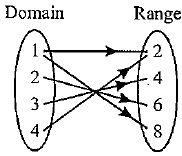Which is not the graph of a function?
To identify any graph, weather it is a function or not, we must understand its definition once again but in terms of graphical meaning.
First condition of function says that – “All the elements of A should be mapped with the elements of B”. That means graphically, for every input in its domain, function must give or provide the corresponding output.
Second condition of the function says that – “Elements of set A should be uniquely mapped with the elements of set B”. These means that, for any input x, we must have one and only one output. The best way to check this condition for the function y = f(x), is draw a line parallel to y – axis. If it cuts the graph at two or more distinct points, this means, for one value of x, we are getting more than one outputs. And hence it will not be a function.

Example
y = x3
Here all the straight lines parallel to y – axis cut y = x3 only at one point.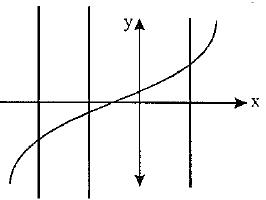Example
x2 + y2 = 1
Here line parallel to y –axis is intersecting the circle at two points hence it is not a function.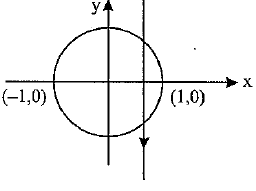Even Function: Let f(x) be a real valued function of a real variable. Then f is even if the following equation holds for all x and -x in the domain of f:
f(x) = f(-x)
Geometrically, the graph of an even function is symmetric with respect to the y-axis.

Odd Function: Again, let f(x) be a real valued function of a real variable. Then f is odd if the following equation holds for all x and -x in the domain of f:
f (-x) = - f(x) or f(x) + f(-x) = 0.

Increasing function: A function f is said to be increasing if whenever a >b, then
f (a) = f(b). Further a function is said to be strictly increasing if
When a > b, then f(a) > f(b). The graph of an increasing function looks somewhat like this: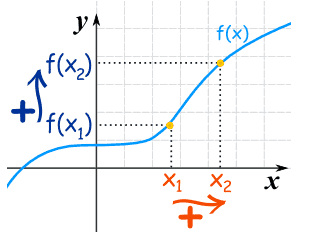You may also refer the video on increasing function

What are the Classification of functions?
Functions are classified as follows:

• Polynomial Function: If a function f is defined by

F(x) = anxn + an-1xn-1 + ...... +ax + a0,

where n is a non negative integer and an, an-1, …, a, a0 are real number and an ≠ 0,  then f is called a Polynomial Function of degree n. A polynomial function is always continuous.

• Algebraic Function: A function f is called an algebraic function if it can be constructed using algebraic operations such as addition, subtraction, multiplication, division and taking roots, started with polynomials

Example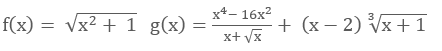Note: All the polynomials are algebraic but converse is not true. Functions which are not algebraic, are known as Transcidental Function.

• Fractional Rational Function: A rational function is a function of the form,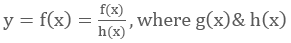are polynomials & h(x) ≠ 0. The domain of f(x) is set of real x such that h(x) ≠ 0.

Example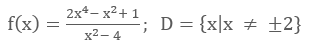• Exponential Function: A function f(x) = ax = exlna(a > 0, a ≠ 1, x ∈ R) is called an Exponential Function. f(x) = ax is called an exponential function because the variable x is the exponent. It should not be confused with power function. g(x) = x2 in which variable x is the base. For f(x)= ex domain in R and range is R+.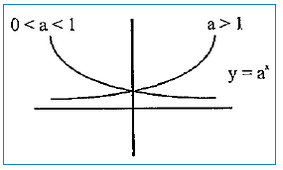• Logarithmic Function: A function of the form y=loga⁡ x, x > 0, a > 0, a ≠ 1, is is called Logarithmic Function.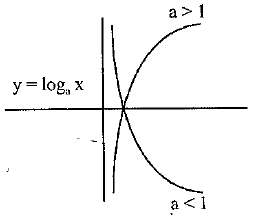• Absolute Value Function (or Modulus Function): A function y= f(x) = |x| is called the Absolute Value Function or Modulus Function. It is defined as: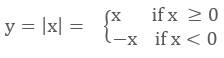For f(x) = |x|,domain is R and Range is R+ U {0}.See below for its figure.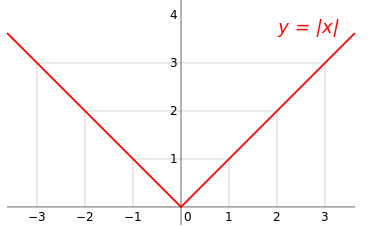• Signum Function: A function y= f(x) = sgn (x)  is defined as follows: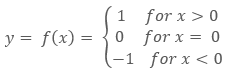It is also written as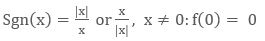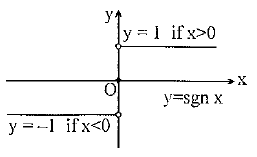• Greatest Integer Or Step up Function: The function y = f(x) = [x] is called the greatest integer function where [x] denotes the greatest integer less than or equal to x. Note that for:
-1 ≤ x <0; [x] = -1
0 ≤ x <1;  [x] = 0
1 ≤ x < 2; [x] = 1
2 ≤ x < 3; [x] = 2 and so on.
For f(x) = [x], domain is R and range is I.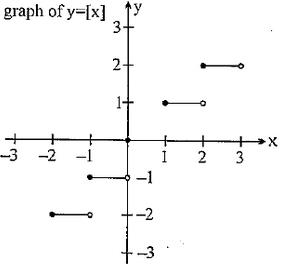• Identity Function: The f: A → B defined by f(x) = x, ∀ x ∈A is called the identity of A and is denoted by IA. The domain and range of identity function is entire real range i.e. R  f(x) = x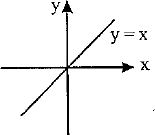Constant Function: The function f: A → B  is said to be a constant function if every element of A has the same f image in B. Thus f : A → B; f(x) = c,∀ x ∈ A, c ∈ B is a constant function. Note that the range of a constant function is a singleton and a constant function may be one – one or many – one, onto or into.

Example
f(x) = [{x}]; g(x) =sin2⁡x + cos2⁡x; h (x) = sgn (x2- 3x + 4) etc, all are constant functions.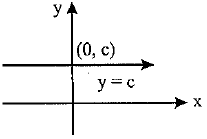Offer running on EduRev: Apply code STAYHOME200 to get INR 200 off on our premium plan EduRev Infinity!

58 docs

,

,

,

,

,

,

,

,

,

,

,

,

,

,

,

,

,

,

,

,

,

;